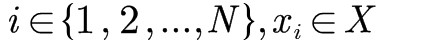# How do I represent this in CVX?

Where does your variable X appear in the expression?xi is a member of vector X.Everything is constant except for xi

Each summand is a rational function of the form \frac{x}{cx+d} so you just need to reduce it algebraically to the form p+\frac{q}{cx+d} and that is p+q*inv_pos(cx+d). You have to assume the domain is such that the denominator is positive and that the function you get is convex/concave in the desired direction. https://docs.mosek.com/modeling-cookbook/cqo.html#rational-functions